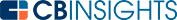# What is Gross Margin Ratio?Gross margin ratio measures the profitability of products — how much income each product generates after paying for its cost. It is calculated by dividing gross margins by net sales.

Gross margin ratio helps companies determine how profitable their inventory and services are and allows them to calculate how much of the revenue is left for paying operating expenses.

## What are the components of a gross margin ratio?

Gross margin ratio takes into consideration the cost of sold goods alone. This is because its primary purpose is measuring the profits from selling products or services.

Gross margin ratio is made up of several important variables:

• Gross profit (revenue – COGS)
• Net sales (gross sales – returns or refunds)
• COGS (cost of goods sold)

The cost of goods sold is the direct labor and material costs for creating products.

## Why is gross margin ratio important?

Gross margin ratio is one of the most effective ways to evaluate the financial performance of a business. This measure reflects productivity in terms of how a company uses its raw materials, labor, and other factors of production.

Since it reveals the amount of profit made by a company after calculating the cost of products sold, it helps to reveal its financial strength and status. It also helps companies to make financial projections and future plans.

## How do you calculate gross margin ratio?

To calculate a company’s gross margin ratio, you can use the following formula:

Gross margin ratio = (total revenue - cost of goods sold (COGS)) / total revenue

To calculate gross margin as a percentage, you can use the following formula:

Gross margin percentage = (total revenue – cost of goods sold) / total revenue x 100

### Why is gross margin ratio important?

Gross margin ratio is one of the most effective ways to evaluate the financial performance of a business. This measure reflects productivity in terms of how a company uses its raw materials, labor, and other factors of production.

Since it reveals the amount of profit made by a company after calculating the cost of products sold, it helps to reveal its financial strength and status. It also helps companies to make financial projections and future plans.

## Gross margin ratio example

Say a company’s revenue is \$100,000 and the cost of goods sold is \$25,000. You can work out the company’s gross margin with the following equation:

\$100,000 - \$25,000 = \$75,000

To work out its gross margin ratio, you can use the following equation:

\$75,000 / \$100,000 = 0.75

This shows that the company’s gross margin ratio is 0.75 or 75%. In other words, each product the company makes generates a revenue of \$0.75 from every dollar. That revenue is what the company uses to pay overheads and expenses.

## What is a good gross margin ratio?

A range of factors can influence the gross margin ratio. It will also be different from one type of business to another. For example, service businesses often have much higher ratios than product-based businesses, because the cost of goods sold is often lower.

A higher gross margin ratio indicates that a company will be able to pay its operating expenses and will probably have profit left over.

## How can a business increase its gross margin ratio?

If a business has a low gross margin ratio, it may need to increase it in order to stay afloat. There are two ways to do so:

## Is gross margin ratio and profit margin ratio the same?

No. Gross margin ratios tell a company how much revenue it has left to pay for regular expenses after paying to produce the goods and services they sell. On the other hand, profit margin ratios tell a company how much revenue is left as profit after all COGS and expenses are paid.

In our previous example, the company had a 75% gross margin ratio, or \$0.75 left from every dollar earned. That income must be used to pay for expenses like employee salaries and other overheads involved with running a business. Once all expenses are added up, there may be a mere \$0.05 left as profit. This would mean that the company’s profit margin ratio is only 5%.

## What are the limitations of gross margin ratios?

Gross margin ratio has some limitations. The biggest is that it isn’t a good yardstick for measuring total profitability. A company’s real profits can only be calculated once all costs are subtracted. These may include costs such as advertising, interest, taxes, labor, material, and overhead costs.

It also isn’t useful for comparing against other industries, as the cost structure and profit determinations vary. The COGS for a restaurant will vary widely from a home health service, for instance. It’s therefore important to compare profitability with similar companies when using this calculation.

Gross margin ratio is simply a calculation that tells a company how much revenue remains after paying for the creation of its goods or services. Companies with high gross margin ratios stand a better chance of having higher profit margins overall. When the cost of goods sold is kept low in comparison to the amount of revenue those products generate, a higher percentage of each dollar of revenue can pay for general expenses and overheads.

You may also like# www.cbinsights.com